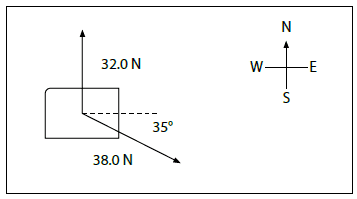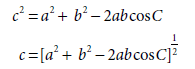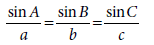# Calculating the net force acting on object in diagram

Evangeline101

## Homework Statement

Calculate the net force acting on each object in the following diagrams. Show your work.## Homework Equations[/B]## The Attempt at a Solution

c2= a2 + b2 - 2abcosC
c = (a2 + b2 - 2abcosC) 1/2

c = [ (32.0 N)2 + (38.0 N)2 - 2 (32.0 N) x (38.0 N) cos 55° ] 1/2
c = 32.8 NsinA/ 38.0 N = sin55° / 32.8 N

A= 72°

Fnet = 32.8 N [ N 72° E]

Can someone please check my answer and tell me if it is correct? Thanks.